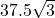## A regular hexagon has sides of 5 feet. What is the area of the hexagon? 12.5 ft 2 37.5 ft 2 25 ft 2 50 ft 2

Question

A regular hexagon has sides of 5 feet. What is the area of the hexagon? 12.5 ft 2 37.5 ft 2 25 ft 2 50 ft 2

in progress 0
5 months 2021-08-09T08:22:26+00:00 1 Answers 8 views 0

1. Answer:This value is exact. We can write this as 37.5*sqrt(3)

This approximates to roughly 64.9519

The units for the area are in square feet.

==========================================

Explanation:

Split the regular hexagon into 6 identical equilateral triangles.

Each equilateral triangle has side length x = 5 ft.

The exact area of one of the equilateral triangles is

A = 0.25*sqrt(3)*x^2

A = 0.25*sqrt(3)*5^2

A = 0.25*sqrt(3)*25

A = 0.25*25*sqrt(3)

A = 6.25*sqrt(3)

Multiply this by 6 to get the exact area of the regular hexagon.

6*A = 6*6.25*sqrt(3) = 37.5*sqrt(3) which is the exact area in terms of radicals or square roots.

If your teacher meant to say choice B is 37.5*sqrt(3), then that would be the final answer. If your teacher only said 37.5 without the sqrt(3) term, then there’s a typo.